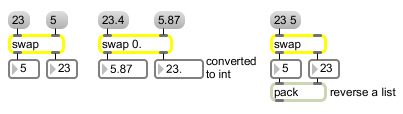# swap

Reverse the sequential order of two integers

## Description

swap swaps the values of its inlets, preserving right-to-left ordering. The first outlets (and second inlets) type follow the argument (or is an int if you give no argument.) The second outlets (and first inlets) type is int or float depending on whether you use the name "swap" or "fswap".

## Arguments

Name Type Opt Description
initial-value/number-format int or float opt Sets an initial value for the number that is to be sent out the left outlet. Float argument will cause a float to be sent out the left outlet. (The number sent out the right outlet is always an int.) If there is no argument, the initial value is 0.

## Messages

 bang In left inlet: Swaps and sends out the numbers currently stored in swap. int input [int] In left inlet: The number is sent out the right outlet, then the number in the right inlet is sent out the left outlet. (inlet1) input [int] In right inlet: The number is stored to be sent out the left outlet when a number is received in the left inlet. float input [float] The numbers are converted to int, unless there is a float argument, in which case the number received in the right inlet is stored as a float. (inlet1) input [float] In right inlet: The number is stored to be sent out the left outlet when a number is received in the left inlet. list input [list] In left inlet: The numbers are stored in swap. The first number is sent out the right outlet, then the second number is sent out the left outlet.

## Output

float: If there is a float argument, the number sent out the left outlet is a float.
int: When a number is received in the left inlet, the number in each inlet is sent out the opposite outlet.

## ExamplesNumbers are sent out in reverse order from that in which they were received